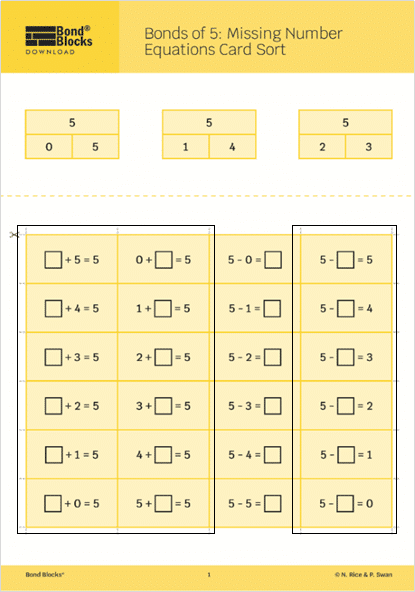## 14) Representing Addition and Subtraction

Bonds of 5: Thinkboard

### Mathematics

Develop the concept of addition and subtraction using Bond Blocks, diagrams, an equation, pictures and word story.

Develop fluency recalling a difficult to remember Bond of 5.

### Language

• bond
• part-part-whole
• subtraction## Differentiation

### A little easier

The teacher completes one thinkboard for a selected two-part bond of five. Students use this as a model, repeating the teacher’s thinkboard, applying it to a different two-part bond of five.

When modelling word problems refer to the “Solving Word Problem” Teacher Notes in regards to modelling a variety of problem types. Students typically find active problems easier than static ones and Part-Part-Whole problems easier than comparison problems. Ensure students are exposed to a range of problem types for both addition and subtraction.

##### Build a repertoire

Encourage students to share their thinkboards with each other so as students can build a repertoire of word stories and representations of the operation. Students can then adapt their friends’ examples by changing the nouns (eg cars for dogs) or the numbers (a different bond of 5). Changing the nouns is easier than changing the numbers.

##### Act it out

Assist students to understand the problem by instructing them them to act out of the equation before representing it with pictures and words. Students can act out the problem with:

• Actual objects (for example, toy cars) that feature in their problem.
• Objects such as blocks that represent the actual object. This is more difficult than using actual objects.

### A little harder

##### Create word questions

Instruct students to create:

• A word question on a blank piece of paper, for another student to answer, and
• A matching answer page for the question they have written. Use the Bonds of 5: Representing Addition Thinkboard activity board as a template for the answer page.

Students can be encouraged to create word questions where

• There are more than two parts.
• The unknown is not in the answer position.
• Support students to create these questions by clicking to download the Bonds of 5: Missing Number Equations Card Sort from the previous activity. Use cards that have equations written where the missing number is not in the answer position. Students can select a card, represent the answer on the Thinkboard and then use this to write a matching word question.

Students can then swap and solve each other’s word questions.### Progression

Repeat the Bonds of 5: Representing Addition Thinkboard activity using subtraction

Activity 14

Bonds of 5: Representing Subtraction Thinkboard.

After students have represented their own addition and subtraction stories they can solve written word problems. Go to

Activity 15

Bonds of 5: Word Problems, Wholes to 5